Next: Bra-ket notation Up: Linear Operators Previous: Basis functions

### Expansion in terms delta function normalized functions

In many applications it is convenient to express a physical amplitude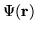not as an expansion of normalized basis functions (as in Eq. (), but in terms of the following functions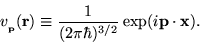(1.18)

Note that the integral,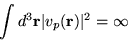(1.19)

is undefined, and so these functions do not represent true physical states. Nevertheless they are useful since, according to Fourier theory, any physical amplitudecan be expressed in terms of them, i.e.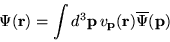(1.20)

where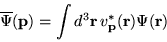(1.21)

is the Fourier transform of. Now according to definition (),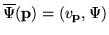and inserting into () we get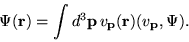(1.22)

This relation is similar to that given by Eq. (), indeed if we consider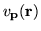to be a basis function then the above relation is expansion (), except for the replacement of the discrete index i, and its sum, with that of a continuous index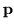and an integral. (Actually, in this example, I used three continuous indices,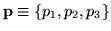and three integrals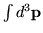. Using the definition of the delta function we find,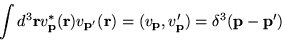(1.23)

a generalization of the normalization conditions exhibited by the basis functions ui discussed above. The functionsare said to obey delta function normalization.

We can construct a table, analogous to the one given for the basis functions ui, that is appropriate for the improper basis functions. pt
 improper basis functions, or vectorsdelta function normalizationState expansion in basisExpansion coefficients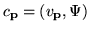Closure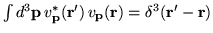Next: Bra-ket notation Up: Linear Operators Previous: Basis functions
Bernard Zygelman
1999-09-21